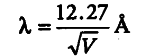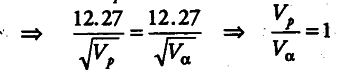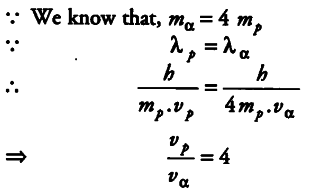# A proton and an a-particle have the same de-Broglie wavelength

A proton and an a-particle have the same de-Broglie wavelength. Determine the ratio of
(i) their accelerating potentials
(ii) their speeds.

(i) The de-Broglie wavelength of a particle is given[where,V is the accelerating potential of the particle](ii) The de-Broglie wavelength of a particle is given
λ = h / mv
{ λ }_{ p } = h / mp . vp and
{ λ }_{ ∝ } = h / { m }_{ ∝ }

{ v }_{ ∝ }